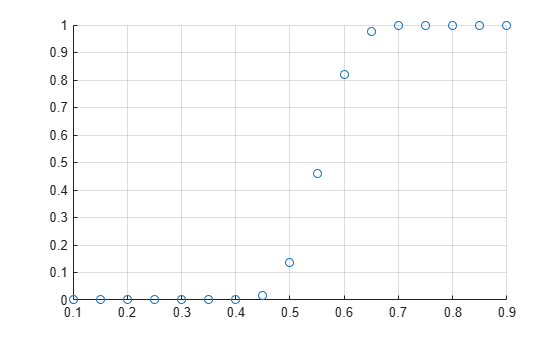# binocdf

Binomial cumulative distribution function

## Syntax

``y = binocdf(x,n,p)``
``y = binocdf(x,n,p,'upper')``

## Description

example

````y = binocdf(x,n,p)` computes a binomial cumulative distribution function at each of the values in `x` using the corresponding number of trials in `n` and the probability of success for each trial in `p`.`x`, `n`, and `p` can be vectors, matrices, or multidimensional arrays of the same size. Alternatively, one or more arguments can be scalars. The `binocdf` function expands scalar inputs to constant arrays with the same dimensions as the other inputs.```

example

````y = binocdf(x,n,p,'upper')` returns the complement of the binomial cumulative distribution function at each value in `x`, using an algorithm that computes the extreme upper tail probabilities more accurately than the default algorithm.```

## Examples

collapse all

Compute and plot the binomial cumulative distribution function for the specified range of integer values, number of trials, and probability of success for each trial.

A baseball team plays 100 games in a season and has a 50-50 chance of winning each game. Find the probability of the team winning more than 55 games in a season.

```format long 1 - binocdf(55,100,0.5)```
```ans = 0.135626512036917 ```

Find the probability of the team winning between 50 and 55 games in a season.

`binocdf(55,100,0.5) - binocdf(49,100,0.5)`
```ans = 0.404168106656672 ```

Compute the probabilities of the team winning more than 55 games in a season if the chance of winning each game ranges from 10% to 90%.

```chance = 0.1:0.05:0.9; y = 1 - binocdf(55,100,chance);```

Plot the results.

```scatter(chance,y) grid on```Compute the complement of the binomial cumulative distribution function with more accurate upper tail probabilities.

A baseball team plays 100 games in a season and has a 50-50 chance of winning each game. Find the probability of the team winning more than 95 games in a season.

```format long 1 - binocdf(95,100,0.5)```
```ans = 0 ```

This result shows that the probability is so close to 1 (within `eps`) that subtracting it from 1 gives 0. To approximate the extreme upper tail probabilities better, compute the complement of the binomial cumulative distribution function directly instead of computing the difference.

`binocdf(95,100,0.5,'upper')`
```ans = 3.224844447881779e-24 ```

Alternatively, use the `binopdf` function to find the probabilities of the team winning 96, 97, 98, 99, and 100 games in a season. Find the sum of these probabilities by using the `sum` function.

`sum(binopdf(96:100,100,0.5),'all')`
```ans = 3.224844447881779e-24 ```

## Input Arguments

collapse all

Values at which to evaluate the binomial cdf, specified as an integer or an array of integers. All values of `x` must belong to the interval ```[0 n]```, where `n` is the number of trials.

Example: `[0 1 3 4]`

Data Types: `single` | `double`

Number of trials, specified as a positive integer or an array of positive integers.

Example: `[10 20 50 100]`

Data Types: `single` | `double`

Probability of success for each trial, specified as a scalar value or an array of scalar values. All values of `p` must belong to the interval `[0 1]`.

Example: `[0.01 0.1 0.5 0.7]`

Data Types: `single` | `double`

## Output Arguments

collapse all

Binomial cdf values, returned as a scalar value or an array of scalar values. Each element in `y` is the binomial cdf value of the distribution evaluated at the corresponding element in `x`.

Data Types: `single` | `double`

collapse all

### Binomial Cumulative Distribution Function

The binomial cumulative distribution function lets you obtain the probability of observing less than or equal to x successes in n trials, with the probability p of success on a single trial.

The binomial cumulative distribution function for a given value x and a given pair of parameters n and p is

`$y=F\left(x|n,p\right)=\sum _{i=0}^{x}\left(\begin{array}{c}n\\ i\end{array}\right){p}^{i}{\left(1-p\right)}^{\left(n-i\right)}{I}_{\left(0,1,...,n\right)}\left(i\right).$`

The resulting value y is the probability of observing up to x successes in n independent trials, where the probability of success in any given trial is p. The indicator function ${I}_{\left(0,1,...,n\right)}\left(i\right)$ ensures that x only adopts values of 0,1,...,n.

## Alternative Functionality

• `binocdf` is a function specific to binomial distribution. Statistics and Machine Learning Toolbox™ also offers the generic function `cdf`, which supports various probability distributions. To use `cdf`, specify the probability distribution name and its parameters. Alternatively, create a `BinomialDistribution` probability distribution object and pass the object as an input argument. Note that the distribution-specific function `binocdf` is faster than the generic function `cdf`.

• Use the Probability Distribution Function app to create an interactive plot of the cumulative distribution function (cdf) or probability density function (pdf) for a probability distribution.